• +91 9971497814
• info@interviewmaterial.com

# Chapter 10 Gravitation Interview Questions Answers

### Related Subjects

Question 1 : State the universal law of gravitation

The universal law of gravitation states that every object in the universe attracts every other object with a force called the gravitational force. The force acting between two objects is directly proportional to the product of their masses and inversely proportional to the square of the distance between their centers.
For two objects of masses m1 and m2 and the distance between them r, the force (F) of attraction acting between them is given by the universal law of gravitation as: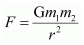Where, G is the universal gravitation constant given by: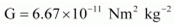Question 2 : Write the formula to find the magnitude of the gravitational force between the earth and an object on the surface of the earth.

Answer 2 : Let ME be the mass of the Earth and m be the mass of an object on its surface. If R is the radius of the Earth, then according to the universal law of gravitation, the gravitational force (F) acting between the Earth and the object is given by the relation:Question 3 : What do you mean by free fall?

Answer 3 : Gravity of the Earth attracts every object towards its centre. When an object is released from a height, it falls towards the surface of the Earth under the influence of gravitational force. The motion of the object is said to have free fall.

Question 4 : What do you mean by acceleration due to gravity?

Answer 4 : When an object falls towards the ground from a height, then its velocity changes during the fall. This changing velocity produces acceleration in the object. This acceleration is known as acceleration due to gravity (g). Its value is given by 9.8 m/s2.

Question 5 : What are the differences between the mass of an object and its weight?

 S. No. Mass Weight I. Mass is the quantity of matter contained in the body. Weight is the force of gravity acting on the body. II. It is the measure of inertia of the body. It is the measure of gravity. III. Mass is a constant quantity. Weight is not a constant quantity. It is different at different places. IV. It only has magnitude. It has magnitude as well as direction. V. Its SI unit is kilogram (kg). Its SI unit is the same as the SI unit of force, i.e., Newton (N).

Question 6 : Why is the weight of an object on the moon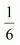th  its weight on the earth?

Answer 6 : Let ME be the mass of the Earth and m be an object on the surface of the Earth. Let RE be the radius of the Earth. According to the universal law of gravitation, weight WE of the object on the surface of the Earth is given by,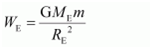Let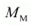and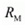be the mass and radius of the moon. Then,according to the universal law of gravitation, weight WM of the object on thesurface of the moon is given by: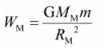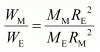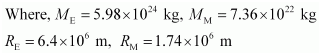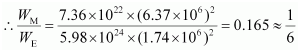Therefore, weight of an object on the moon isof its weight on the Earth.

Question 7 : Why is it difficult to hold a school bag having a strap made of a thin and strong string?

Answer 7 : It is difficult to hold a school bag having a thin strap because the pressure on the shoulders is quite large. This is because the pressure is inversely proportional to the surface area on which the force acts. The smaller is the surface area; the larger will be the pressure on the surface. In the case of a thin strap, the contact surface area is very small. Hence, the pressure exerted on the shoulder is very large.

Question 8 : What do you mean by buoyancy?

Answer 8 : The upward force exerted by a liquid on an object immersed in it is known as buoyancy. When you try to immerse an object in water, then you can feel an upward force exerted on the object, which increases as you push the object deeper into water.

Question 9 : Why does an object float or sink when placed on the surface of water?

Answer 9 : If the density of an object is more than the density of the liquid, then it sinks in the liquid. This is because the buoyant force acting on the object is less than the force of gravity. On the other hand, if the density of the object is less than the density of the liquid, then it floats on the surface of the liquid. This is because the buoyant force acting on the object is greater than the force of gravity.

Question 10 : You find your mass to be 42 kg on a weighing machine. Is your mass more or less than 42 kg?

Answer 10 : When you weigh your body, an upward force acts on it. This upward force is the buoyant force. As a result, the body gets pushed slightly upwards, causing the weighing machine to show a reading less than the actual value.

Todays Deals### Chapter 10 Gravitation Contributorskrishan

Name:
Email:

# Latest News# 9000 interview questions in different categories